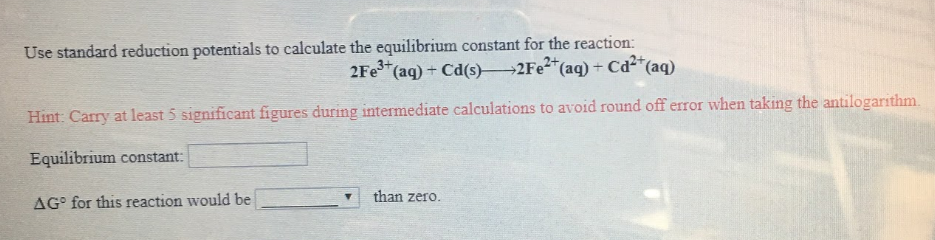# Use standard reduction potentials to calculate the equilibrium constant for the reaction: 2Fe^3+(aq) + Cd(s) → 2Fe^2+(aq) + Cd^2+(aq) Hint: Carry at least 5 significant figures during intermediate calculations to avoid round off error when taking the antilogarithm. Equilibrium constant: ΔG° for this reaction would be ____ than zero.